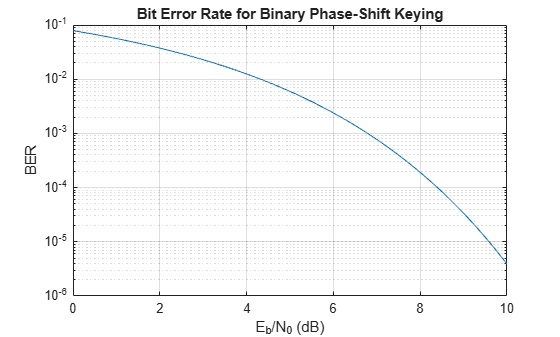Documentation

# erfc

Complementary error function

## Syntax

``erfc(x)``

## Description

example

````erfc(x)` returns the Complementary Error Function evaluated for each element of `x`. Use the `erfc` function to replace `1 - erf(x)` for greater accuracy when `erf(x)` is close to `1`.```

## Examples

collapse all

Find the complementary error function of a value.

`erfc(0.35)`
```ans = 0.6206 ```

Find the complementary error function of the elements of a vector.

```V = [-0.5 0 1 0.72]; erfc(V)```
```ans = 1×4 1.5205 1.0000 0.1573 0.3086 ```

Find the complementary error function of the elements of a matrix.

```M = [0.29 -0.11; 3.1 -2.9]; erfc(M)```
```ans = 2×2 0.6817 1.1236 0.0000 2.0000 ```

The bit error rate (BER) of binary phase-shift keying (BPSK), assuming additive white Gaussian noise (AWGN), is

`${P}_{b}=\frac{1}{2}erfc\left(\sqrt{\frac{{E}_{b}}{{N}_{0}}}\right).$`

Plot the BER for BPSK for values of ${E}_{b}/{N}_{0}$ from `0dB` to `10dB`.

```EbN0_dB = 0:0.1:10; EbN0 = 10.^(EbN0_dB/10); BER = 1/2.*erfc(sqrt(EbN0)); semilogy(EbN0_dB,BER) grid on ylabel('BER') xlabel('E_b/N_0 (dB)') title('Bit Error Rate for Binary Phase-Shift Keying')```You can use the complementary error function `erfc` in place of `1 - erf(x)` to avoid roundoff errors when `erf(x)` is close to `1`.

Show how to avoid roundoff errors by calculating `1 - erf(10)` using `erfc(10)`. The original calculation returns `0` while `erfc(10)` returns the correct result.

`1 - erf(10)`
```ans = 0 ```
`erfc(10)`
```ans = 2.0885e-45 ```

## Input Arguments

collapse all

Input, specified as a real number, or a vector, matrix, or multidimensional array of real numbers. `x` cannot be sparse.

Data Types: `single` | `double`

collapse all

### Complementary Error Function

The complementary error function of x is defined as

`$\begin{array}{c}\text{erfc}\left(x\right)=\frac{2}{\sqrt{\pi }}{\int }_{x}^{\infty }{e}^{-{t}^{2}}dt\\ =1-\text{erf}\left(x\right).\end{array}$`

It is related to the error function as

`$\text{erfc}\left(x\right)=1-\text{erf}\left(x\right).$`

## Tips

• You can also find the standard normal probability distribution using the Statistics and Machine Learning Toolbox™ function `normcdf`. The relationship between the error function `erfc` and `normcdf` is

`$\text{normcdf}\left(x\right)=\left(\frac{1}{2}\right)×\text{erfc}\left(\frac{-x}{\sqrt{2}}\right)$`

• For expressions of the form `1 - erfc(x)`, use the error function `erf` instead. This substitution maintains accuracy. When `erfc(x)` is close to `1`, then `1 - erfc(x)` is a small number and might be rounded down to `0`. Instead, replace `1 - erfc(x)` with `erf(x)`.

• For expressions of the form `exp(x^2)*erfc(x)`, use the scaled complementary error function `erfcx` instead. This substitution maintains accuracy by avoiding roundoff errors for large values of `x`.